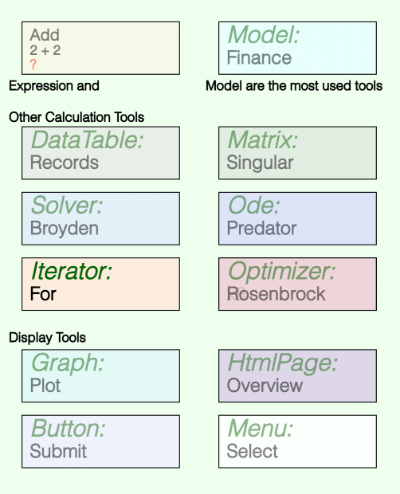The Tools

The built in calculation tools are listed below. To see the help page for a tool, just tap on its name.

• Expression - contains a single formula or constant. For instance if an expression named distance has the formula:

5 km

and an expression named speed has the formula:

distance / 180 s

then speed's value will be 27.78 m/s or 100 kph.

• Data Table - a data base like table with named fields (columns) of different types.
• Function Solver - attempts to solve functions of the form

f(x) = 0

for one or more values of x. That is it attempts to come up with a set of x values such that all the function values are 0.

• Iterator - Math Minion's ability to work with arrays and matrices means traditional programming loops are usually not necessary, but if the need arises, this tool provides a for loop type capability.
• Matrix - while all Math Minion numerical values are in fact matrices, this tool allows you to construct more complex entities, including cell formulas that refer to the values of other cells.
• Model - a model is simply a container for other tools and is what is represented by the diagram view.

All Math Minion sessions have at least one root model, but models can contain other models, so a hierarchy of different models can be constructed.

• ODE Solver (Integrator) - the Ordinary Differential Equation solver is used integrate problems where:

dy/dt = f(y, t)

given an initial value for y, which can be a scalar, array or matrix.

• Optimizer - given some function:

y = f(x)

where y is a scalar and x can be a scalar or an array, the optimizer attempts to come up with the set of x values that produces the smallest value of y.

• Graph - displays a 2D or 3D plot.
• HTML Page - used to create customized input and result views using HTML and javascript.
• Imported Model - a model that is imported from a different session.

Adding a Tool to the Model

To add a tool to the model, tap on the diagram background where you wish the upper left hand of the tool's icon to be placed.

Select Add Tool to Model from the resulting menu and then select the type of tool you wish from the list presented.

Icons

Tools are all represented on the diagram as rectangular icons, with connections being made from the corners of an icon to the middle of a vertical side of all the tools that reference it.

Each type of tool icon has a unique color and all except the expression icon have labels for the tool type and the tool's name. The expression icon, has labels for the tool's name, formula (truncated as necessary) and calculated value. Note the value won't be calculated until the tool is selected or a tool that depends on its value is selected.